# MP6: ECG¶

In this lab we'll try reading in some ECG (electrocardiogram) files, and using the autocorrelation function to find the heartbeat period and filter out noise. You can find the code package, including this file (mp6overview.ipynb), at http://courses.engr.illinois.edu/ece401/fa2020/ece401_20fall_mp6.zip

In :
import numpy as np
import matplotlib.pyplot as plt
import matplotlib.figure
import wave,math
%matplotlib inline

In :
import importlib
import mp6

Out:
<module 'mp6' from '/Users/jhasegaw/Dropbox/mark/teaching/ece401/ece401labs/20fall/mp6/src/mp6.py'>

## Exploring the Data¶

The data directory contains a copy of the MIT-BIH ECG noise stress test database, https://www.physionet.org/content/nstdb/1.0.0/. This database contains two different 30-minute ECG records, each of which has been corrupted by muscle movement noise at six different noise levels. First, let's look at one of the noisy signals.

The wfdb module can be installed using pip install wfdb. The signal object is provided for you in solutions.hdf5, so you only need to install wfdb if you want to read in the raw signals from the distributed files.

In :
import wfdb
nsamps = noisysignal.shape
fig=plt.figure(figsize=(14,4))
axs=fig.subplots(2,1)
axs.plot(time,noisysignal)
axs.plot(time[(time>300)&(time<325)],noisysignal[(time>300)&(time<325),:])

Out:
{'fs': 360,
'sig_len': 650000,
'n_sig': 2,
'base_date': None,
'base_time': None,
'units': ['mV', 'mV'],
'sig_name': ['MLII', 'V1'],
'comments': ["Created by nst' from records 118 and em (SNR = 0 dB)"]}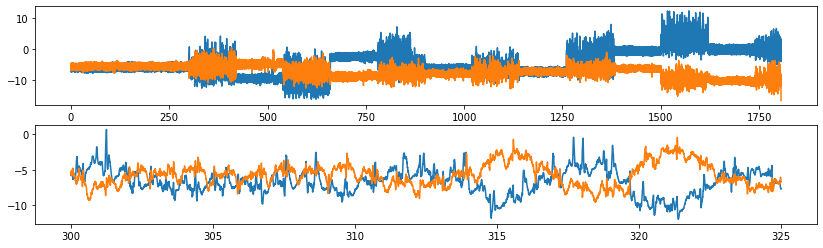Next, let's look at one of the clean signals.

In :
import wfdb
nsamps = cleansignal.shape
fig=plt.figure(figsize=(14,4))
axs=fig.subplots(2,1)
axs.plot(np.arange(0,nsamps),cleansignal)
axs.plot(time[(time>300)&(time<325)],cleansignal[(time>300)&(time<325),:])

Out:
{'fs': 360,
'sig_len': 650000,
'n_sig': 2,
'base_date': None,
'base_time': None,
'units': ['mV', 'mV'],
'sig_name': ['MLII', 'V1'],
'comments': ['69 M 1456 653 x2',
'Digoxin, Norpace',
'The PVCs are multiform.']}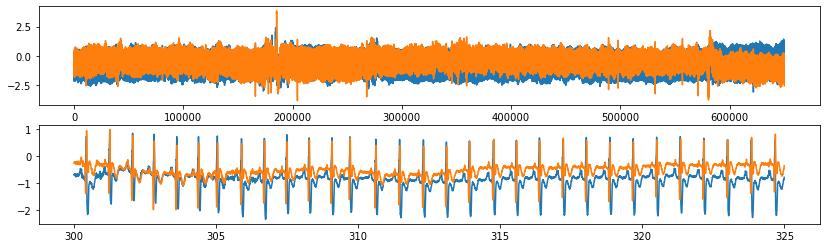## How to debug¶

Rather than printing every plot using separate blocks for both the distributed solution and your solution, instead, each of the following blocks will include lines to plot either one or the other. In order to switch between your solution and the distributed solution, you'll need to comment out the other one.

In :
import h5py
solutions = h5py.File('solutions.hdf5','r')


## Designing the Wiener Filter¶

First, let's design the Wiener filter. We'll do that in three steps:

1. Chop the noisy signal and clean signal into non-overlapping 60-second frames, and compute a power spectrum for each frame.
2. Average the power spectra of the clean and noisy signals, across all frames, in order to simulate calculating expectation. Then divide them to find the Wiener filter's frequency response.
3. Inverse transform to find the Wiener filter's frequency response.
4. Filter the noisy signal, using the Wiener filter. See if it's any cleaner!
In :
importlib.reload(mp6)
frameskip = framelen
#cleanframes,cleanspec=mp6.todo_energyspec(cleansignal,framelen)
#noisyframes,noisyspec=mp6.todo_energyspec(noisysignal,framelen)
cleanframes = solutions['cleanframes']
cleanspec = solutions['cleanspec']
noisyframes = solutions['noisyframes']
noisyspec = solutions['noisyspec']

fig=plt.figure(figsize=(14,6))
axs=fig.subplots(2,2)
frame = 200
axs[0,0].plot(time,cleanframes[frame,:])
axs[0,0].set_title('Clean Frame %d'%(frame))
axs[0,1].plot(freq,20*np.log10(cleanspec[frame,:]))
axs[0,1].set_title('Clean Power Spectrum of Frame %d'%(frame))
axs[1,0].plot(time,noisyframes[frame,:])
axs[1,0].set_title('Noisy Frame %d'%(frame))
axs[1,1].plot(freq,20*np.log10(noisyspec[frame,:]))
axs[1,1].set_title('Noisy Power Spectrum of Frame %d'%(frame))
fig.tight_layout()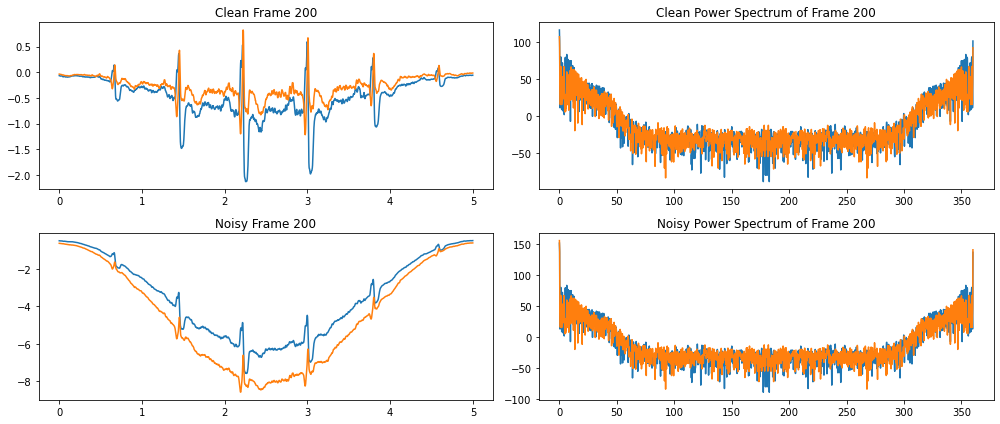Next, we divide the clean spectrum by the noisy spectrum, in order to create the Wiener filter. In the plot below you can see that the Wiener filter is mostly a little bit below 0dB (magnitude=1), with two important exceptions.

1. The response is very small (-50dB) at 0Hz, because the noise signals have a 0Hz offset, while the clean signals do not. This 0Hz offset is a common phenomenon in ECG signals, sometimes called "baseline wander."
2. There is a broad peak, between about 0Hz and 50Hz, because in this band, the signal has more energy than the noise.
3. There are also positive-going spikes once every $F_s/12$ radians or so ($F_s$ is the sampling frequency), suggesting that the signal is periodic with a period of about 12 samples.
In :
importlib.reload(mp6)
print(cleanspec.shape)
print(noisyspec.shape)
#wienerfreq = mp6.todo_wienerfreq(cleanspec, noisyspec)
wienerfreq = solutions['wienerfreq']

fig=plt.figure(figsize=(14,6))
ax=fig.subplots()
ax.plot(freq,20*np.log10(wienerfreq))
#ax.set_ylim(-5,40)

(361, 1800, 2)
(361, 1800, 2)

Out:
[<matplotlib.lines.Line2D at 0x7fb015f31340>]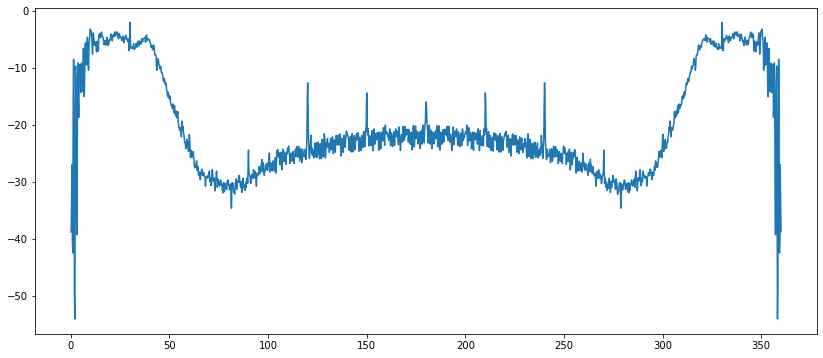Now let's find the impulse response of this filter, by inverse transforming. After inverse transforming, you need to use fftshift to put the $n=0$ sample in the center of the impulse response. By default, ifft computes the output for samples numbered $n=0$ through $n=N-1$, where $N$ is the frame length. What we really want, though, is the impulse response for samples $n=-(N-1)/2$ through $n=(N-1)/2$, i.e., an impulse response that is symmetric in time. Fortunately, fftshift can give that to us, because the FFT is basically a Fourier series: it assumes that the impulse response is periodic in time, so that $h[-1]=h[N-1]$, and $h[-2]=h[N-2]$, and so on.

In :
importlib.reload(mp6)
#wienerfir = mp6.todo_wienerfir(wienerfreq)
wienerfir = solutions['wienerfir']

fig=plt.figure(figsize=(14,6))
ax=fig.subplots()
ax.plot(time, wienerfir)
ax.set_title('Estimated Wiener filter, impulse response')
wienerfir

Out:
<HDF5 dataset "wienerfir": shape (1800,), type "<f8">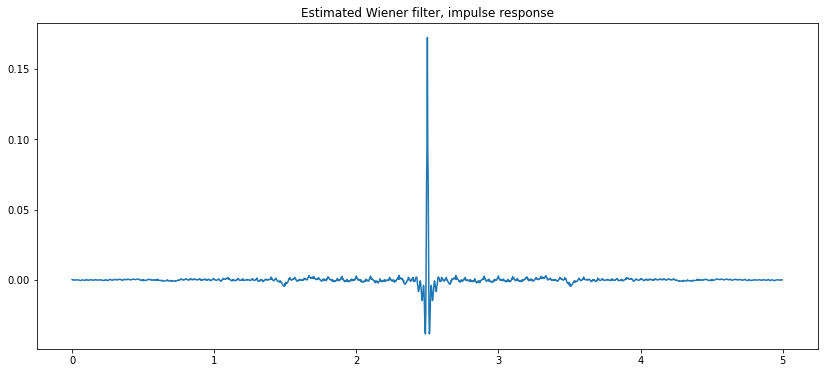OK. Let's try filtering the noisy signal using the Wiener filter, to see if it gets any cleaner!

In :
importlib.reload(mp6)
#outputsignal = mp6.todo_convolve(noisysignal, wienerfir)
outputsignal = solutions['outputsignal']
#outputframes, outputspec = mp6.todo_energyspec(outputsignal,framelen)
outputframes = solutions['outputframes']
outputspec = solutions['outputspec']

fig=plt.figure(figsize=(14,6))
axs=fig.subplots(3,2)
frame = 200
axs[0,0].plot(time,cleanframes[frame,:])
axs[0,0].set_title('Clean Frame %d'%(frame))
axs[0,1].plot(freq,20*np.log10(cleanspec[frame,:]))
axs[0,1].set_title('Clean Power Spectrum of Frame %d'%(frame))
axs[1,0].plot(time,noisyframes[frame,:])
axs[1,0].set_title('Noisy Frame %d'%(frame))
axs[1,1].plot(freq,20*np.log10(noisyspec[frame,:]))
axs[1,1].set_title('Noisy Power Spectrum of Frame %d'%(frame))
axs[2,0].plot(time,outputframes[frame,:])
axs[2,0].set_title('Output Frame %d'%(frame))
axs[2,1].plot(freq,20*np.log10(outputspec[frame,:]))
axs[2,1].set_title('Output Power Spectrum of Frame %d'%(frame))
fig.tight_layout()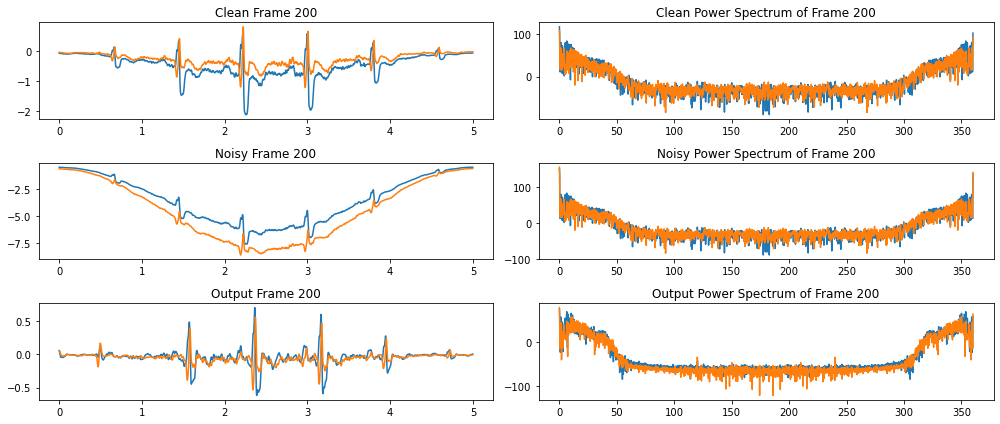## Tracking Inter-Beat Interval¶

Now let's try to track the inter-beat intervals, and perhaps even try to find the locations of each of the heartbeats. First, let's use the outputframes we've already computed, and compute the autocorrelation of each frame.

In :
importlib.reload(mp6)
#autocor = mp6.todo_autocor(outputframes)
autocor = solutions['autocor']

fig=plt.figure(figsize=(14,4))
ax=fig.subplots()
frame=200
ax.plot(autocor[frame,:,:])

Out:
[<matplotlib.lines.Line2D at 0x7fb01c5f1280>,
<matplotlib.lines.Line2D at 0x7fb01c5f13a0>]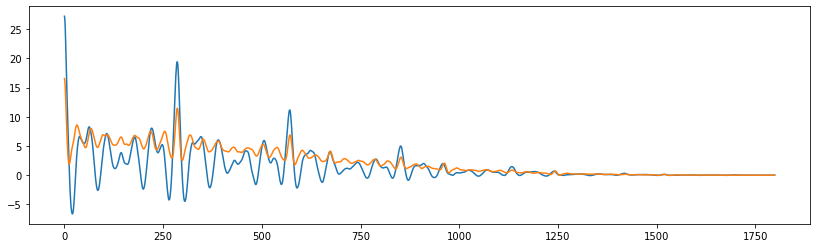Let's find the inter-beat interval for each frame, for each channel, using the cleaned-up signal. To do this, we just find the largest autocorrelation coefficient $r_{yy}[m]$, in the range between about $125\le m\le 1000$. There will still be some noise in the IBIs we calculate this way, but the Wiener filter has removed much of it. In fact, we find that this particular patient, during this 30-minute period, had a pretty steady heartbeat IBI of about 300 samples, a little less than one second.

In :
importlib.reload(mp6)
#ibi =  mp6.todo_ibi(autocor,125,1000)
ibi = solutions['ibi']

fig=plt.figure(figsize=(14,4))
axs=fig.subplots(2,1)
axs.plot(outputsignal)
axs.set_title('Cleaned-up signals $y[n]$')
axs.plot(ibi)
axs.set_title('Estimated inter-beat interval, in samples')
print('Average IBI is %d samp ~ %g sec'%(ibi[0,0],ibi[0,0]/header['fs']))

Average IBI is 301 samp ~ 0.836111 sec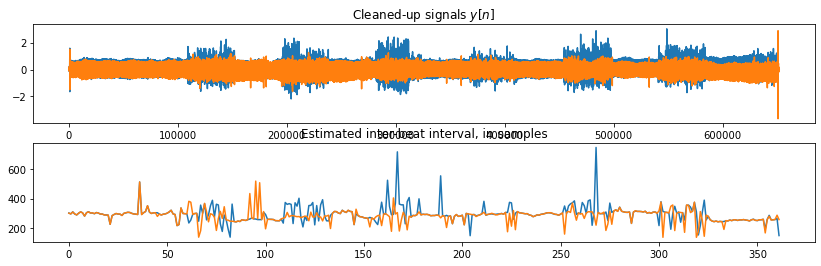Finally, let's locate the individual heartbeats (the QRS pulse) in each frame. There are several ways to do this, but let's use the easy way:

1. Divide the $c$th channel of the $t$th frame into non-overlapping segments of length equal to the IBI.
2. Find $n=$argmax of the signal amplitude in each such segment, and put peaks[t,n,c]=1 in that sample, 0 everywhere else.
In :
importlib.reload(mp6)
#peaks = mp6.todo_peaks(ibi, outputframes)
peaks = solutions['peaks']

frame = 200
fig=plt.figure(figsize=(14,6))
axs=fig.subplots(2,1)
frame = 200
axs.plot(outputframes[frame,:,:])
axs.set_title('Output Frame %d (IBI=%d, %d)'%(frame,ibi[frame,0],ibi[frame,1]))
axs.plot(peaks[frame,:,:])
axs.set_title('Peak Locations of Frame %d'%(frame))
fig.tight_layout()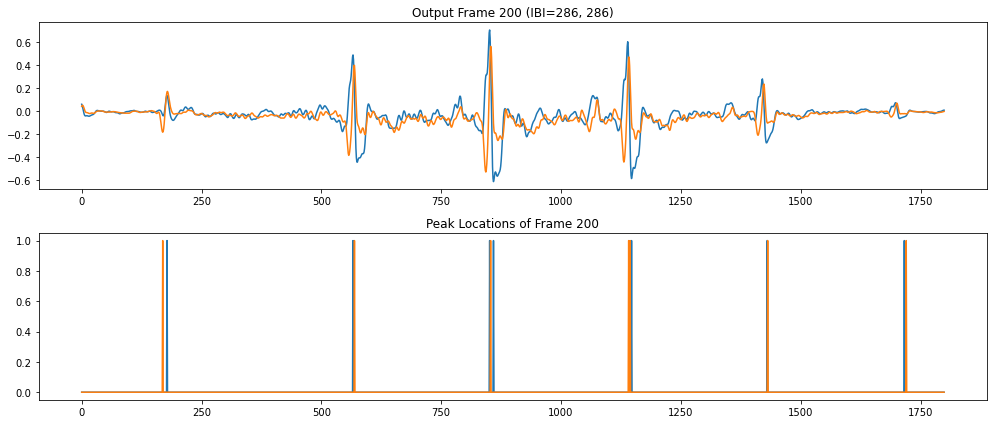Not all frames got cleaned up as well, so not all frames have results that look as pretty:

In :
fig=plt.figure(figsize=(14,6))
axs=fig.subplots(2,1)
frame = 300
axs.plot(outputframes[frame,:,:])
axs.set_title('Output Frame %d (IBI=%d, %d)'%(frame,ibi[frame,0],ibi[frame,1]))
axs.plot(peaks[frame,:,:])
axs.set_title('Peak Locations of Frame %d'%(frame))
fig.tight_layout()
`